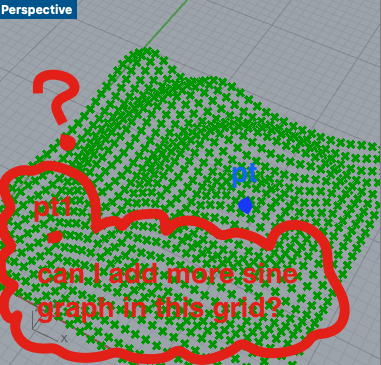# Grasshopper c# - interference sine wave in 3d

Hi!

I am struggling how to make multiple sine graphs in one grid points. In other words, multiple sine waves interfere each other in 3d. Below is my c# script ;

List pts = new List();
for(int j = 0 ; j < v ; j++){
for(int i = 0; i < u ; i++){
Point3d p = new Point3d(i, j, 0);
double dist = pt.DistanceTo§;
double z = Math.Sin(dist);
Vector3d vec = new Vector3d(0, 0, z);
p += vec;
}
}

A = pts;

In my script, the sine graph starts from pt (blue color in the screenshot) and I wish to add one more sine graph starts from pt1(red color in the screenshot image). And the final result will be two sine graph interfere each other.Here attached the source file! How_To_Multiple_pts.gh (6.8 KB)

Related: Ripple/Raindrop Pattern

1 Like

HI
You can use Point3dList.ClosestPointInList() to find the closest point. Then use the nearest point to calculate the vector Z value.

``````List<Point3d> pts = new List<Point3d>();
for(int j = 0 ; j < v ; j++){
for(int i = 0; i < u ; i++){
Point3d p = new Point3d(i, j, 0);
//Find the closest point
Point3d cp = Point3dList.ClosestPointInList(points, p);
double dist = cp.DistanceTo(p);
double z = Math.Sin(dist);
Vector3d vec = new Vector3d(0, 0, z);
p += vec;